﻿ 潜艇单舱大深度破损进水模型数值分析 Numerical Analysis to Inflow Changes Model of Single-Cabin-Broken Submarine in Large Depths

Vol. 08  No. 05 ( 2019 ), Article ID: 30174 , 7 pages
10.12677/AAM.2019.85098

Numerical Analysis to Inflow Changes Model of Single-Cabin-Broken Submarine in Large Depths

Dawei Han*, Dongjun Zhang, Tianzhong Wang, Hongming Li, Lei Zhang

The No. 92337 Troop of PLA, Dalian Liaoning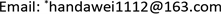Received: Apr. 16th, 2019; accepted: May 7th, 2019; published: May 14th, 2019ABSTRACT

A criterion of the dynamic anti-sinking effectiveness is given based on the pressure-containing ability of the plane bulkhead, and the inflow changes model of single-cabin-broken submarine is established, as well as the calculating process and method of the maximum floatable depth. The influence of the broken area and the vertical speed to the maximum floatable depth is researched by numerical simulation. The results reveal that the maximum floatable depth linearly corresponds well to the vertical speed, and the summary diagram of maximum floatable depths calculating formulas to different vertical speeds is established on the basis of this regular, which can offer support to commander’s rapid decision when the submarine is broken in war.

Keywords:Submarine, Broken, Dynamic Anti-Sinking, Maximum Floatable Depth, Numerical Analysis1. 引言

2. 潜艇单舱大深度破损动力抗沉有效性准则

2.1. 潜艇战时大深度损管操纵原则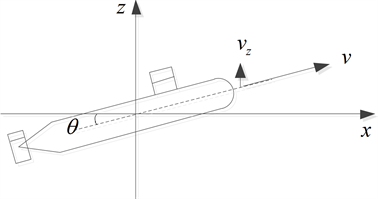Figure 1. Vertical motion of the submarine

$d{v}_{z}=d\left(v\mathrm{sin}\theta \right)$ (1)

2.2. 潜艇单舱大深度破损动力抗沉有效性判据

$t\le {t}_{cr}$ (2)

3. 潜艇单舱大深度破损进水模型

3.1. 潜艇单舱大深度破损进水微分方程

${P}_{1}=\frac{{P}_{o}{V}_{o}}{{V}_{o}-V}$ (3)

$\frac{\text{d}V}{\text{d}t}=\mu A\sqrt{2g\left(H-{h}_{b}-\frac{10{V}_{o}}{{V}_{o}-V}+10\right)}$ (4)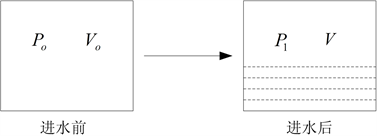Figure 2. Pressure-change after the water entering the cabin

3.2. 潜艇单舱大深度破损进水差分方程

$\frac{\text{d}V}{\text{d}t}=\frac{{V}_{n+1}-{V}_{n}}{\Delta t}$ (5)

$\frac{\text{d}H}{\text{d}t}=\frac{{H}_{n+1}-{H}_{n}}{\Delta t}=-{v}_{z}$ (6)

${V}_{n+1}=\Delta t\cdot \mu A\sqrt{2g\left({H}_{n}-\Delta t{v}_{z}-\frac{10{V}_{o}}{{V}_{o}-{V}_{n}}+10\right)}+{V}_{n}$ (7)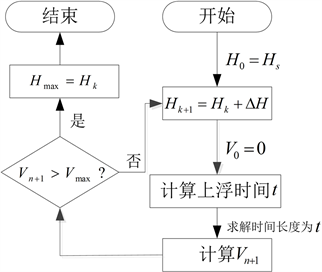Figure 3. Numerical calculating flow diagram

4. 数值解法算例及分析

4.1. 破口面积的影响Figure 4. Maximum floatable depth to different broken areas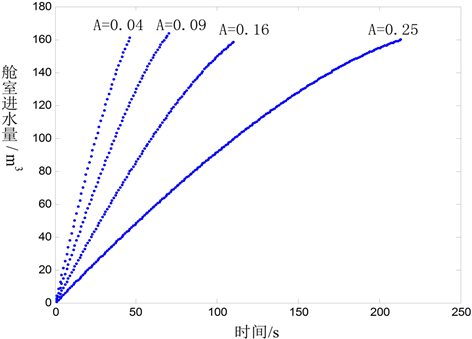Figure 5. Inflow changes to different broken areas

4.2. 潜艇升速的影响

${H}_{\mathrm{max}}=148{v}_{z}+46.4$ (8)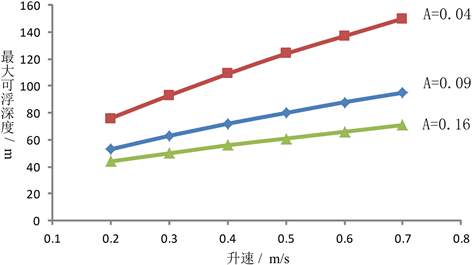Figure 6. Maximum floatable depths to different vertical speeds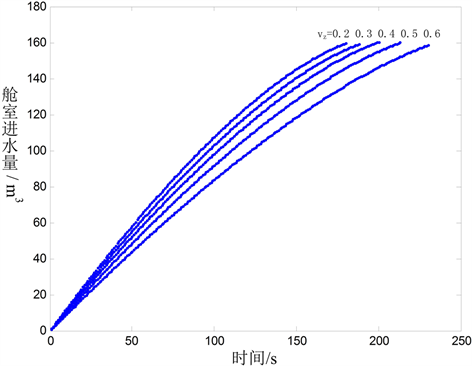Figure 7. Inflow changes to different vertical speeds

4.3. 不同破口面积不同升速下潜艇最大可浮深度查询计算Table 1. Maximum floatable depths calculating formulas to different vertical speeds of submarine

5. 结论

Numerical Analysis to Inflow Changes Model of Single-Cabin-Broken Submarine in Large Depths[J]. 应用数学进展, 2019, 08(05): 876-882. https://doi.org/10.12677/AAM.2019.85098

1. 1. 刘常波, 刘蓁, 黄海峰. 高压气吹除对潜艇动力抗沉影响分析研究[J]. 舰船电子工程, 2015, 35(1): 91-98.

2. 2. 徐亦凡, 陈丘岳, 胡坤. 高压气吹除时机对潜艇动力抗沉影响的仿真研究[J]. 舰船电子工程, 2010, 30(11): 114-115, 124.

3. 3. 张建华, 胡坤, 徐亦凡. 航速对破损潜艇动力抗沉的影响分析[J]. 舰船科学技术, 2010, 32(1): 51-53.

4. 4. 王京齐. 潜艇的承载力与水下动力抗沉[J]. 海军工程大学学报, 2003, 15(4): 65-68, 72.

5. 5. 朱军. 潜艇动力抗沉性能评估研究[J]. 船舶工程, 2003, 25(4): 43-46.

6. 6. 林俊兴, 戴余良, 张涛, 黄华斌. 潜艇承载力分析和动力抗沉操纵方法研究[J]. 海军工程大学学报, 2004, 16(2): 27-29.

7. 7. 陈水泉, 朱军, 曾广会. 潜艇动力抗沉运动特性分析[J]. 海军工程大学学报, 2010, 22(1): 51-61.